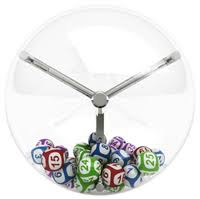## Thursday, 26 January 2012

### When Is Random Really Random?A surprising amount of what we do in computer security relies upon the use of random numbers and yet not many of us actually take the time to think about how these numbers are being generated.  We blithely assume that our computer, when required, can generate a truly random number.  But wait! How can a machine that works in a deterministic manner generate something that is truly random.  The simple answer is that it can't.  The best we can do is to generate a number that is pseudorandom.

The fact that we rely upon pseudorandom numbers is a potential problem for IT security. After all, if a machine is using a known algorithm to generate a number that your system then treats as random, what is to stop an attacker from calculating that same number if he knows your algorithm. It is a fundamental truth of any strong computer security that you must assume that an attacker knows the algorithms that you have used. So, perhaps one should spend a little more time understanding how pseudorandom numbers are generated in order that they are not relied upon inappropriately.

The measure of random in a number is known as entropy.  Not the entropy as physicists use it, but entropy as cryptographers use it.  Entropy is, in essence, how uncertain you are about a number.  This means that the entropy is not necessarily related to the number of bits in a number but instead to the number of possible values that number could have taken.  Imagine being able to discard the number of bits in a number about which you were certain.  The number of bits that remain are the number of bits of entropy. Suppose, for example, a 100 bit number could take on two possible values, then you only need one bit to differentiate those two values.  Hence, such a number would be described as having 1bit of entropy.  Obviously this is an extreme case, and I have not complicated the issue by factoring in the different types of probability distribution.Most mathematicians tend to define the entropy of a number X as:

H(X) ≔ − ∑P(X=x) log₂P(X=x)

where the summation is from 0 to a number x, and P(X=x) is the probability of X taking the value x.

So, how do you generate a number that has to greatest possible entropy. There has been much research on using activities such as mouse movements or keystrokes to generate a number that can be considered random.  But so far it appears that the activities picked to generate the numbers have proved to be not quite as random as first thought: some typists are remarkably accurate and consistent!  Other work has focused on incorporating some form of physical device into a computer that can generate truly random numbers based upon provably chaotic behaviour.  This has two drawbacks: generating large random numbers can take an unacceptably long time, and the device might fail rendering the numbers predictable, without you knowing.Which brings us back to the generation of pseudorandom numbers.  Most accept that this is the best method of generating numbers with sufficient entropy, whilst making the mechanism efficient and accessible enough for widespread use in computing.  There are many algorithms in the literature but I would recommend an approach such as described in Fortuna which was an improvement by Ferguson, Schneier and Koho on a previous method called Yarrow.  Fortuna, like most approaches, relies upon a seed  which is a relatively small but truly random number.  But, it allows for the use of modern encryption techniques such as AES which mean that although the algorithm is known it is highly unlikely that the resulting pseudorandom number sequence can be reproduced.  What Fortuna shows is that rather than rely upon standard libraries on their own, you construct a routine that, yes, uses standard libraries, but recognises the potential attacks against those and seeks to minimise the threat by incorporating elements such as AES and SHA thereby reserving some secret information to the local machine, decreasing the chances that a third party could reproduce your number(s).So, in short, the best way to use random numbers is by generating pseudrandom numbers but in such a way that "unreliable" sources of randomness are replaced by effectively creating your own store of entropy upon which you can draw and keeping the means by which that store is generated secret by virtue of a secret key.

For a superb, free book that goes into great depth about random number generation, see Luc Devroye's book here (originally published with Springer-Verlag, New York, 1986).